﻿ Discussion on Mass in a Gravitational Field

### Discussion on Mass in a Gravitational Field

Zhan Likui, Sun YiningOPEN ACCESSPEER-REVIEWED

## Discussion on Mass in a Gravitational Field

Zhan Likui1,, Sun Yining1

1Chinese Academy of Science, HeFei, China

### Abstract

In a black hole, no force can prevent matter from collapsing into a point, a gravitational singularity. The problem of the singularity is always a difficult one. We attempt to discuss gravitation from another angle in this work. Based on experimental analysis and theoretical verification, a hypothesis regarding mass in gravitational fields is presented. It can avoid the problem of singularity, and the meaning of gravitation is simple to determine.

### At a glance: Figures

12
Prev Next

• Likui, Zhan, and Sun Yining. "Discussion on Mass in a Gravitational Field." International Journal of Physics 1.5 (2013): 110-114.
• Likui, Z. , & Yining, S. (2013). Discussion on Mass in a Gravitational Field. International Journal of Physics, 1(5), 110-114.
• Likui, Zhan, and Sun Yining. "Discussion on Mass in a Gravitational Field." International Journal of Physics 1, no. 5 (2013): 110-114.

 Import into BibTeX Import into EndNote Import into RefMan Import into RefWorks

### 1. Introduction

In theory, a gravitational singularity should have zero volume and infinite density. Scientists have made various attempts to uncover the secrets of the singularity. Today, much breakthrough has been made in this area of research, and a black hole does not mean absolutely darkened [1, 2]. Even so, the truth of black holes has yet to be confirmed [3, 4]. The evidence of and remain to be answered . After various attempts, we propose a way of thinking about gravitation from energy perspective. For simplicity, the nouns and terms in the work conform to the definitions of fundamental physics. The “clock” is a timepiece in the physical sense; some examples include an atomic clock, a mechanical clock, a quartz clock or another chronometric instrument, excluding a gravity pendulum which cannot work in outer space. The “ruler” is a length-measuring tool in the physical sense; some examples include a meter stick or another instrument that measures dimensions. “Mass” is inertial mass, an object measured by its resistance to acceleration.

### 2. Methods

2.1. Annihilation Test

Experiment 1: On the ground of a celestial body, antimatter and matter could be used to annihilate one another. They could be brought into contact gradually and transformed into photons. All of the energy would be measured by an energy meter “E” in outer space. This scenario is labeled with an “a” in Figure 1. Finally, a certain amount of energy, E1, would be obtained.

Experiment 2: Equal amounts of antimatter and matter could be weighed. They could be kept separate and lifted out of the gravitational field by an elevator. Then, the matter and antimatter could be brought together in outer space. This scenario is labeled with a “b” in Figure 1. This time, a certain amount of energy, E2, would be obtained.

Figure 1. Repeated annihilation would cause a gravitational field to disappear. If annihilation were to occur on the ground, the energy that the photons lost would not be found (a). If annihilation were to occur in outer space, the energy of the external work would not be found (b)

When a light from a gravitational field streams into outer space, frequency of the light is reduced, it is called gravitational redshift. The reason of the redshift is often considered as the gravitational field pulls the photons . In other words, the photons transfer part of their energy to the gravitational field in the process. Theoretically, experiment 1 can be repeated continuously. Ultimately, all of the mass available on the body would be depleted. (This is, of course, only a thought experiment, and given antimatter and matter attracts each other . The gravitational field should receive a significant amount of energy. However, the gravitational field would disappear with the body. The lost energy could not be found.

Similarly, experiment 2 would be also repeated indefinitely. Finally, all the matter and the gravitational field would disappear. Lifting the objects out of the gravitational field requires a third party to provide external work. The energy of the external work could not be found.

2.2. Acceleration Test

Compared with a clock period in outer space, the clock period within a radius R of a gravitational field is defined by the following relation .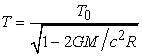(2.1)

where T0 is the clock period in outer space, G is the gravitational constant, c is the speed of light, and M is mass of the celestial body.

The standard ruler is defined by the speed of light. In view of the speed of light is a constant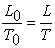(2.2)

where L0 is length of the standard ruler in outer space and L is its length within the radius R of the gravitational field.

we can obtain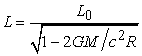(2.3)

Experiment 3: In outer space, a force F was exerted on a mass m for duration T0 (labeled with an “a” in Figure 2). The displacement is represented by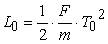(2.4)

Experiment 4: The test was staged on the ground along a smooth horizontal plane (labeled with “b” in Figure 2). The displacement would be given by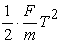(2.5)
Figure 2. An acceleration test is staged in outer space (a) and on the ground (b). In both places, the clock and the ruler would be variable and the mass would be constant, but different results would be observed

Given the Equation (2.1)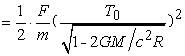(2.6)

Given the Equation (2.3) and (2.4)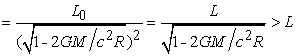(2.7)

Different results would be observed in the both places. However, there is no evidence to indicate that Newton's second law takes a different form at different altitudes, even if the relativistic effect was taken into account .

2.3. Energy in a Gravitational Field

in view of above antinomies, the following hypotheses are necessary:

ⅰ). Energy cannot be transferred between a photon and a gravitational field.

ⅱ). Mass is variable in a gravitational field.

In Figure 1, if the mass in the gravitational field and in outer space are expressed as m and m0, the external work is expressed as W, the following equations can be obtained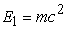(3.1)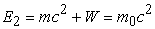(3.2)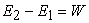(3.3)

In the case of the reverse process, a meteoroid is captured by the gravitational field, it accelerates toward the ground, and finally, the meteoroid hits the ground, and gives off light and heat. If the meteoroid does not lose any mass, considering the increased energy, light and heat, when a star collapses and becomes a black hole (a naked singularity with zero radius), its mass should increase infinitely, which would be disastrous. In fact, the gravitational potential energy of all matter in the gravitational field also has been increased by the addition of the meteoroid.

The gravitational redshift must result from the light source, mass of atoms of the light source has reduced in the gravitational field, rather than the gravitational field pulls photons. That a ray of light can be changed in direction by a massive object should not be interpreted as that the photo is pulled by the object, if so, delay of the radar echo cannot be explained . Furthermore, a photo can be accelerated or decelerated disagrees with the constancy of the speed of light.

In experimental measurements of the gravitational redshift using the Mossbauer Effect , the radiation source is placed at the top of a tower, and the absorber is placed on the ground. Photons can thus be absorbed. Now, an operator takes the absorber and ascends to the top of the tower. However, photons cannot be absorbed. This is why? We know that quantum field theory states that photons and particles exist in the vacuum . And cosmic microwave background radiation (2.7 K) fills the cosmic space. If energy of the photons can increase by a gravitational field, a black hole should grow stronger continuously without any matter, which is hard to understand.

Thus, the external work transfers to the lifted object where in Figure 1. In reference to special relativity, after external work W is done to an object of mass m, the final mass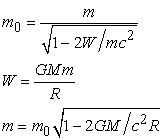(3.4)

This relationship describes the difference in the mass of an object when in a gravitational field versus in outer space.

2.4. Covariance in a Gravitational Field

Lorentz covariance is a key property of space-time that follows from the special theory of relativity; the covariance requirement states that physical laws take the same form in any inertial coordinate system. For example, in a high-speed spacecraft, rulers become shorter and clocks tick more slowly, objects increase in mass. If the mass were to remain constant, an object would be easier to accelerate in the spacecraft than where on the ground. Thus, we can determine the absolute velocity of the spacecraft by this. However, all of the attempts to explore absolute velocity have failed, and time slows down in a high-speed system has been proved by experiments . In the high-speed spacecraft, where clocks tick more slowly, and rulers become shorter. In other words, spectrum of atoms in the spacecraft has a lower frequency and a shorter wavelength. An observer in the spacecraft observes the same color just as we do on the earth. The relativity principle is reliable.

From some perspective, mass refers to the rest mass, and everything else is kinetic energy. In fact, kinetic energy only has relative significance, if another frame of reference is specified, and is different from that previously specified. For example, kinetic energy of the earth in the universe cannot be confirmed. Change of mass has been validated in many ways, for example, high-speed electrons have greater inertia mass in a cyclotron.

Einstein theorized that physical laws take the same form in all reference frames in general relativity . Today, many laser interferometers are located around the world for detecting gravitational waves. They have improved the precision of the Michelson-Morley experiments to an unprecedented degree. The earth travels in the universe and with a 24-hour cycle of rotation. In view of the sun influences the intensity of the gravitational field where the laboratories . However, no report has stated that the result has to do with the rotation period of the earth. In view of various accurate experiments , for example, experiments of gravitational mass and inertial mass, experiments of the speed of light, etc [14, 15]. There is no evidence that different forms of physical law are needed at different altitudes, even outer space. In actuality, this idea has been recognized.

Given a centripetal force of a circular motion in outer space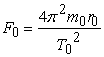(4.1)

where r0 is the radius of the circular motion, T0 is the period, and m0 is the mass of the particle.

In a horizontal plane in a gravitational field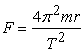(4.2)

where parameters within a radius R of a gravitational field do not contain a subscript ‘0’.

Given the Equation (2.1), (2.3) and (3.4), we obtain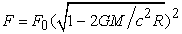(4.3)

Equation (2.4) should be revised as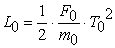(4.4)

Given the Equation (3.4), (4.3) and (4.4), Equation (2.5) can be solved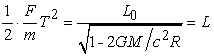(4.5)

Thus, Newton's second law takes the same form.

When an electron enters a gravitational field, its mass decreases, the charge-mass ratio remains unchanged according to . The quantity of the elementary charge should also be reduced. A proton does likewise. In other words, the bonding force between a proton and an electron are weakened in a gravitational field, which can explain the origin of the gravitational redshift, and the size of an object increases in a gravitational field. Put another way, a spring dynamometer should be a little softer in a gravitational field. This is why wavelengths of a light source become unlimited length in the Schwarzschild radius.

2.5. Mercury's Perihelion Advance

Of all the planets in the solar system, Mercury is the closest to the sun, and changes in length and mass cannot be ignored. Namely, the weaker the gravitational field, the more precise Newton's theory will be. Let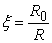(5.1)

where R is the true orbit radius of a planet and R0 is the expectation.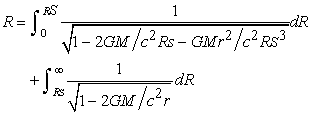(5.2)

where RS is the radius of the body of the gravitational field.

This equation can be solved numerically with a computer. We could give the approximate solution here. The length of a ruler in the gravitational field is shown in Figure 3.

Figure 3. A ruler in a gravitational field: L- True length, L0 - expected length, LH - length of the ruler at one-half of the orbit radius

It can be proved according to Gauss’s theorem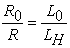(5.3)

where LH is the length of the ruler at one-half of the orbit radius.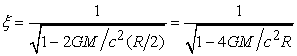(5.4)

The description of mass can also be deduced by the same method. The modified parameters can applied to the equation of complete square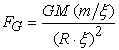(5.5)

The equation can be expanded, and negligibly small terms are ignored: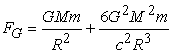(5.6)

The parameter FG is substituted into the Binet equation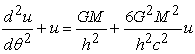(5.7)

where u=1/R and h are the moment of momentum of unit mass.

The differential equations is easy to solve, and the precession of the perihelion after one revolution is given by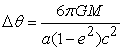(5.8)

where a is the semimajor axis of the orbit, and e is the orbital eccentricity.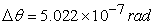In a century, Mercury revolves around the sun 4.1521 times. Thus, the additional is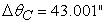2.6. Delay of Radar Waves

In 1964, Shapiro, an American scholar, made radar waves pass the edge of the Sun. They reached Venus on the other side of the Sun and were reflected back to Earth. The actual time of travel was more than 200 microseconds longer than expected .

An observer outside the gravitational field uses his or her own clock and ruler to measure the speed of light at a radius R of the gravitational field. In view of the difference of the clocks and rulers, the result is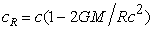(6.1)

The course of the radar waves is divided into two parts, and the actual time is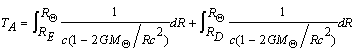(6.2)

where RE is the radius of Earth's orbit and RD is the radius of Venus’s orbit.

The expected time is thus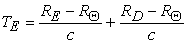(6.3)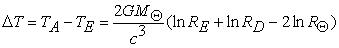(6.4)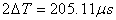(6.5)

In fact, this kind of phenomenon can be seen often. A ray of light penetrates a piece of glass, it slows down, because people use the same clock and ruler outside the glass instead of where in the glass. Similarly, the deflection of light beside a heavenly body can be solved in the same way.

### 3. Discussion

Einstein has suggested that an experiment is conducted in a free-falling elevator or in outer space which is indistinguishable. However, experiments have confirmed that a clock ticks more slowly in a gravitational field than it in outer space. The reason is that two identical clocks are used, and they are placed in different spaces to accomplish this. To be more precise, the only difference between the two clocks is the external work. Clocks in a local scope have no difference in the external work. The concept of external work can help us to understand the space-time curvature. The reason of the twin paradox should be that one of the twins has been applied a lot of external work, as the situation that a clock is applied external work in a gravitational field. In this way, an instrument big enough is good for detecting the gravitational waves.

Heisenberg uncertainty principle has been proclaimed that a particle with greater mass occupy smaller space, which is the theoretical basis of electron microscopes. De Broglie successfully extended the wave-particle duality of photons to the microcosmic field, and a particle with greater energy has a shorter wavelength. High-energy photons have a shorter wavelength. Particle physicists usually use mass to extrapolate the size of particles . Overall, a heavier particle has a smaller size in the microscopic world.

Usually, a larger stone is heavier, but in the microscopic world, the opposite is true. However, there is also evidence of the same phenomenon in the macroscopic world, for example, when an object is moving at a high speed, its size decreases, and its mass increases; when an object enters a gravitational field, its size increases, and its mass decreases. This change is a reflection of the microcosmic scale. In this sense, mass should be variable in a gravitational field.

We try to understand gravitation. An object enters a gravitational field can release energy. The space in the gravitational field has a lower energy state, and any particle will always jump toward the lower energy state and release energy, that is, gravitation.

When an object changes in mass m and gravitational potential energy U in a gravitational field, it can be proven that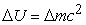(7)

It could be called Mass-Field relationship (MF), and the typical proof is the gravitational red shift. The ΔU can be determined by the external work. Theoretically, any point in the universe can be regarded as the reference such as the ground, as long as the relative external work of the destination location can be calculated. However, outer space is more representative than where in a gravitational field, in which a clock and other apparatus remain unchanged, for example, the edge of the Galaxy is more representative than that of the Solar System, sending a satellite there need more external work.

An experiment to verify the mass-field relationship can be a consideration. In Figure 4, two copper balls are fixed on both sides of an axis. The balls in the device, labeled “a,” rotate in the horizontal plane; balls in another device, labeled “b,” rotate in the vertical plane. They run in vacuum chamber on the ground, and are driven and receive the same initial velocity. S is a double-decked superconducting magnetic bearing used to monitor the energy loss. The direction of the axis of device b is perpendicular to the earth's axis (east-west direction) such that the directions of the axes have the same change in a day.

Figure 4. Balls rotate around an axis in the horizontal plane (a) and in the vertical plane (b). The gravitational potential energy of the balls in device b changes continuously

Based on this fact, any transformation of different forms of energy must take time, which is the theoretical basis of the clock. From the standpoint of the mass-field relationship, the height of the balls in device b changes continuously, which causes transformations between the mass and the gravitational potential energy. A delayed change of mass is equivalent to attaching a resistance to device b. lastly, the rotation of device b would lag behind that of device a.

It is worthwhile studying whether the mass-field relationship is a universal relationship, such as the electromagnetic force, the weak force and the nuclear force, which might explain the origin of the mass defect and even provide a way to construct a universal theory, for example, physical and chemical properties of matter in a strong electric field or a strong magnetic field or the borders of the fields. For example, a strong electric field is applied to the receptor of a Mossbauer spectroscope to study the change of the atomic energy level. Confirming the mass-field relationship might generate additional ideas about the current questions in physics [17, 18, 19].

### 4. Conclusions

Based on the above study, equal amounts of matter and antimatter experience complete annihilation, the gravitation field disappears, and energy that the photons lost cannot be found. The principle of the constant speed of light implies a photon could not be accelerated or decelerated. A black hole could not grow stronger indefinitely in the cosmic microwave background. Thus, hypothesis (i) that energy cannot be transferred between a photon and a gravitational field is tenable.

An object that is taken away from a gravitational field requires a third party to provide energy. Newton's second law takes the same form, and time and length are variable between on the ground and in outer space. A gravitational singularity cannot have infinite mass. Therefore, hypothesis (ii) that mass is variable in a gravitational field is tenable. The tow hypotheses can mutually confirm each other, and can resolve some issues regarding gravitational field satisfactorily and consistent with the fundamental principle of general relativity.

### References

  S. W. Hawking, Particle Creation by Black Holes, Commun. Math. Phys. 43, 3, 199, (1975).In article  Maulik K. Parikh, Frank Wilczek, Hawking radiation as tunnelling, Phys. Rev. Lett., 85, 5042 (2000).In article CrossRef  T. Vachaspati, D. Stojkovic, and L. M. Krauss, Observation of incipient black holes and the information loss problem, Phys. Rev. D 76, 024005 (2007).In article CrossRef  Wald Robert M, General Relativity, (University of Chicago Press, 1984).In article CrossRef  Mannheim, P. D., Alternatives to Dark Matter and Dark Energy, Prog. Part. Nucl. Phys. 56, 2, 340 (2006).In article CrossRef  Zeng Qingzhang, Cui Shizi, Relativity and space-time, (Shanxi Science and Technology Press, 1999) [In Chinese].In article  S. Pakvasa, W. A. Simmons, and T. J. Weiler, Test of equivalence principle for neutrinos and antineutrinos, Phys. Rev. D 39, 1761 (1989).In article CrossRef PubMed  Wang Renchuan, Introduction to General Relativity, (University of Science and Technology of China Press 1996) [In Chinese].In article  Irwin I. Shapiro, Testing General Relativity with Radar, Phys. Rev. 141, 1219-1222 (1966).In article CrossRef  T. E. Cranshaw and J. P. Schiffer, Measurement of the gravitational redshift with the Mossbauer effect, Proc. Phys. Soc., 84, 245 (1964).In article CrossRef  P. A. M. Dirac: Principles of Quantum Mechanics, (Oxford university press, 1958).In article  D. H. Frisch and J. B. Smith, Measurement of the Relativistic Time Dilation Using Muons, Am. J. Phys. 31, 5, 342 (1963).In article  S. Weinberg, Gravitation and Cosmology, (John Wiley, New York, 1972).In article  S Schlamminger, K..-Y. Choi, T. A. Wagner, J. H. Gundlach, and E. G. Adelberger, Test of the Equivalence Principle Using a Rotating Torsion Balance, Phys. Rev. Lett. 100, 041101 (2008).In article CrossRef PubMed  Liang-Cheng Tu et al, The mass of the photon, Rep. Prog. Phys. 68, 77 (2005).In article CrossRef  R. Eisberg and R. Resnick, Quantum Physics of Atoms, Molecules, Solids, Nuclei and Particles, (John Wiley and Sons, 2nd ed. 1985).In article PubMed  G. Vedrenne, Jean-Luc Atteia, The brightest explosions in the Universe, (Springer Berlin Heidelberg New York 2009).In article  P. Laurent, J. Rodriguez, J. Wilms, M. Cadolle Bel, K. Pottschmidt, and V. Grinberg, Polarized Gamma-Ray Emission from the Galactic Black Hole Cygnus X-1, Science. 332, 6028 (2011).In article CrossRef PubMed  D. J. Mortlock, et al, A luminous quasar at a redshift of z = 7.085, Nature 474, 616-619 (2011).In article CrossRef PubMed